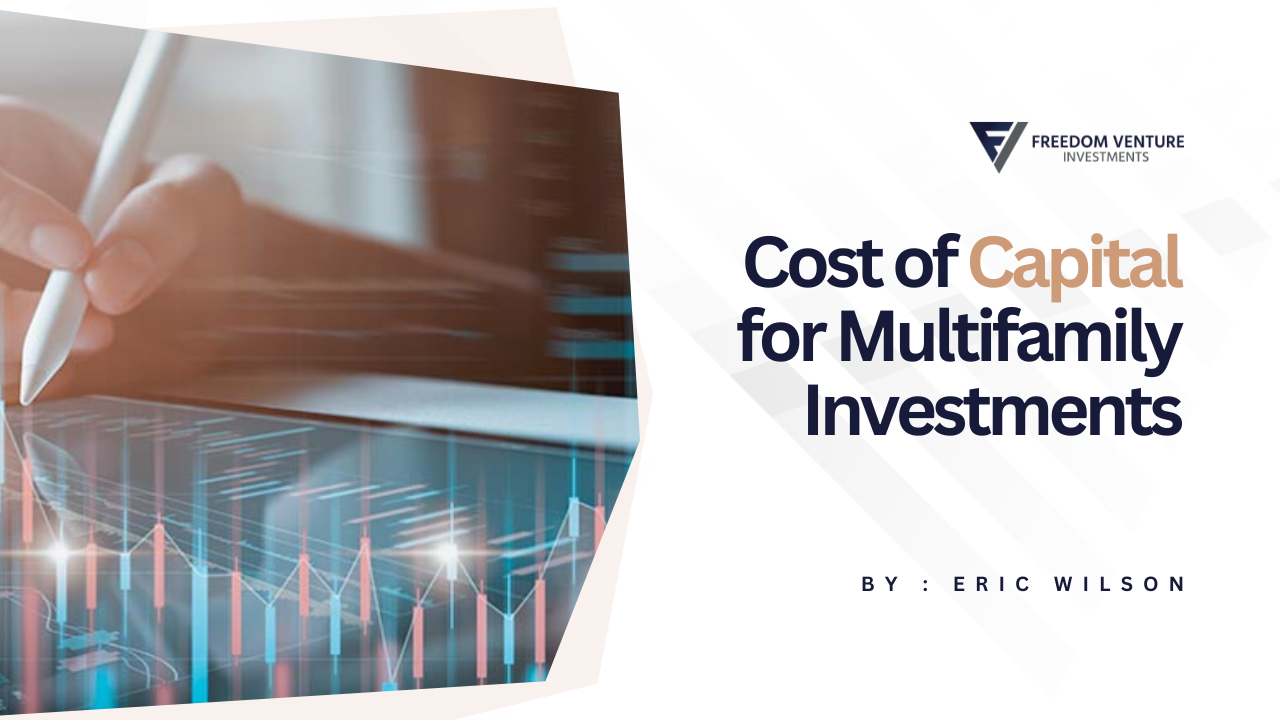### Home### Resources## Cost of Capital for Multifamily Investments### Eric Wilson

COO

May 1, 2023### Eric Wilson

COO

May 1, 2023Multifamily real estate can offer an attractive risk-adjusted return for investors, but it's crucial to understand the cost of capital involved.

### The Cost of Capital: A Basic Primer

The cost of capital is the minimum rate of return that a business or an investment must earn before generating value. In the context of multifamily real estate, it refers to the cost of financing the acquisition or development of a property, which could include bank loans, private loans, or equity contributions.### Cost of Equity and Cost of Debt

The cost of capital consists of the cost of equity and the cost of debt.

### 1. Cost of Equity:

The cost of equity is the return required by an investor to justify the risk of investing capital. It represents the compensation the market demands in exchange for owning the asset and bearing the risk. The cost of equity for a multifamily real estate investment could be derived from the expected return on investment (ROI) by investors.

### 2. Cost of Debt:

The cost of debt is essentially the interest paid on the borrowed money. It's typically lower than the cost of equity due to the lower risk associated with debt. Debt is repaid before equity in the case of a liquidation event, which makes it less risky for the lender.

### Weighted Average Cost of Capital (WACC)

The Weighted Average Cost of Capital (WACC) is a calculation of a company's or an investment's cost of capital that considers each category of capital proportionately. In a multifamily investment, this would include both equity and debt.

WACC can be used as the discount rate in a Discounted Cash Flow (DCF) analysis to determine the net present value (NPV) of future cash flows, which is crucial when evaluating potential investments.

### How Cost of Capital Influences Multifamily Investments

The cost of capital plays a significant role in determining the profitability of a multifamily real estate investment:

1. Investment Analysis: Understanding the cost of capital helps investors determine whether a potential investment is worthwhile. If the expected return on an investment is greater than the cost of capital, it signifies that the investment is likely to generate value.
2. Financing Decisions: The cost of capital can influence the mix of debt and equity used to finance an investment. Typically, a lower cost of capital makes an investment more attractive, influencing investors to lean more towards that source of financing.
3. Risk Assessment: A higher cost of capital typically indicates a higher perceived risk. Understanding this can help investors assess the risk-reward trade-off of an investment.

### Conclusion

Understanding the cost of capital is fundamental to making sound investment decisions. It plays a pivotal role in assessing the potential return, risk, and value of an investment. By comprehensively understanding this concept, you can make well-informed decisions that align with your investment goals in the multifamily real estate market.